﻿ GPS-LEO无线电中性大气掩星反演误差分析文章快速检索 高级检索
 大地测量与地球动力学2018, Vol. 38Issue (11): 1159-1164  DOI: 10.14075/j.jgg.2018.11.012### 引用本文ZHANG Jiman, LIN Jian, ZHU Fuying. Retrieval on GPS-LEO Atmospheric Radio Occultation and Error Sources Analysis[J]. Journal of Geodesy and Geodynamics, 2018, 38(11): 1159-1164.### Foundation support

Director Fund of Institute of Seismology, CEA, No.IS201716161;The Spark Program of Earthquake Technology of CEA, No.XH18032.

### About the first author

ZHANG Jiman, postgraduate, majors in the GNSS meteorology, E-mail:skyzhang181@gmail.com.

### 文章历史

GPS-LEO无线电中性大气掩星反演误差分析

1. 中国地震局地震研究所(地震大地测量重点实验室)，武汉市洪山侧路40号，430071;
2. 中国地震局地壳应力研究所武汉科技创新基地，武汉市洪山侧路40号，430071

1 GPS-LEO掩星反演基本流程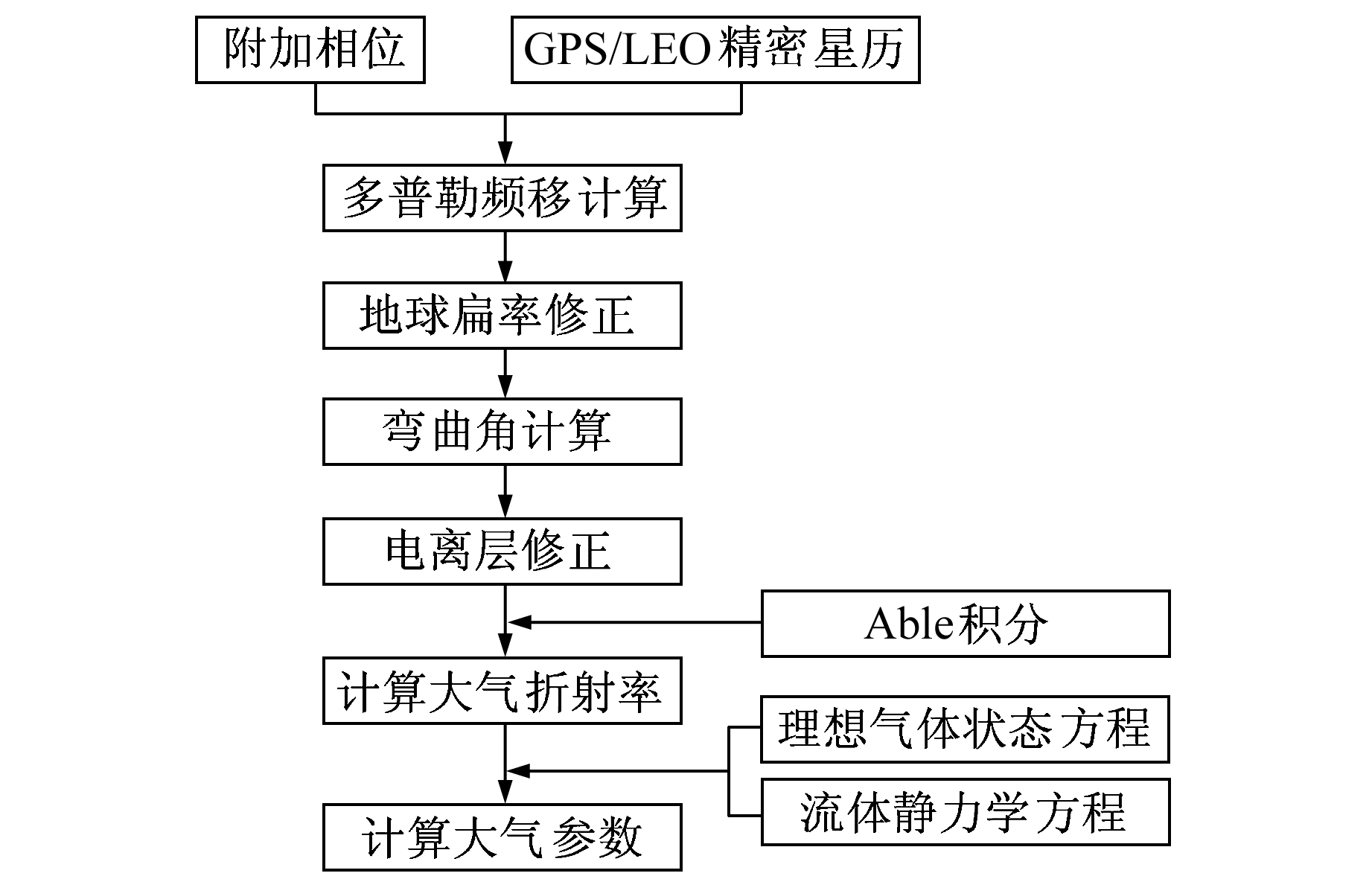图 1 GPS-LEO掩星反演基本流程 Fig. 1 Retrieval processing for GPS-LEO radio occultaion

 $\frac{{\Delta {f_i}}}{{{f_i}}} = \frac{1}{c}\frac{{{\rm{d}}{\varphi _i}}}{{{\rm{d}}t}},i = 1,2$ (1)

 $\Delta {f_i} = \frac{{{f_i}}}{c}\left[ {{\mathit{\boldsymbol{v}}_{\rm{L}}}{{\mathit{\boldsymbol{\bar T}}}_{\rm{G}}} - {\mathit{\boldsymbol{v}}_{\rm{G}}}{{\mathit{\boldsymbol{\hat T}}}_{\rm{L}}} - \left( {{\mathit{\boldsymbol{v}}_{\rm{L}}}{{\mathit{\boldsymbol{\hat r}}}_{{\rm{LG}}}} - {\mathit{\boldsymbol{v}}_{\rm{G}}}{{\mathit{\boldsymbol{\hat r}}}_{{\rm{LG}}}}} \right)} \right]$ (2)

 $n\left( a \right) = \exp \left( {\frac{1}{\pi }\int_a^\infty {\frac{{{\alpha _{{\rm{stat}}}}\left( \xi \right)}}{{\sqrt {{\xi ^2} - {a^2}} }}{\rm{d}}\xi } } \right)$ (3)

2 仿真模拟表 1 仿真数据设置 Tab. 1 Simulation data settings

3 误差分析 3.1 接收机钟误差

 ${y_n} = \sqrt 6 \tau _s^{ - 0.5}{A_{1{\rm{sec}}}}{\rm{ran}}{{\rm{d}}_n}$ (4)
 $\Delta {L_n} = c{\tau _s}{y_n} + \Delta {L_{n - 1}}$ (5)表 2 小量级钟稳定性影响设定及结果 Tab. 2 Minor level clock stability influence settings and results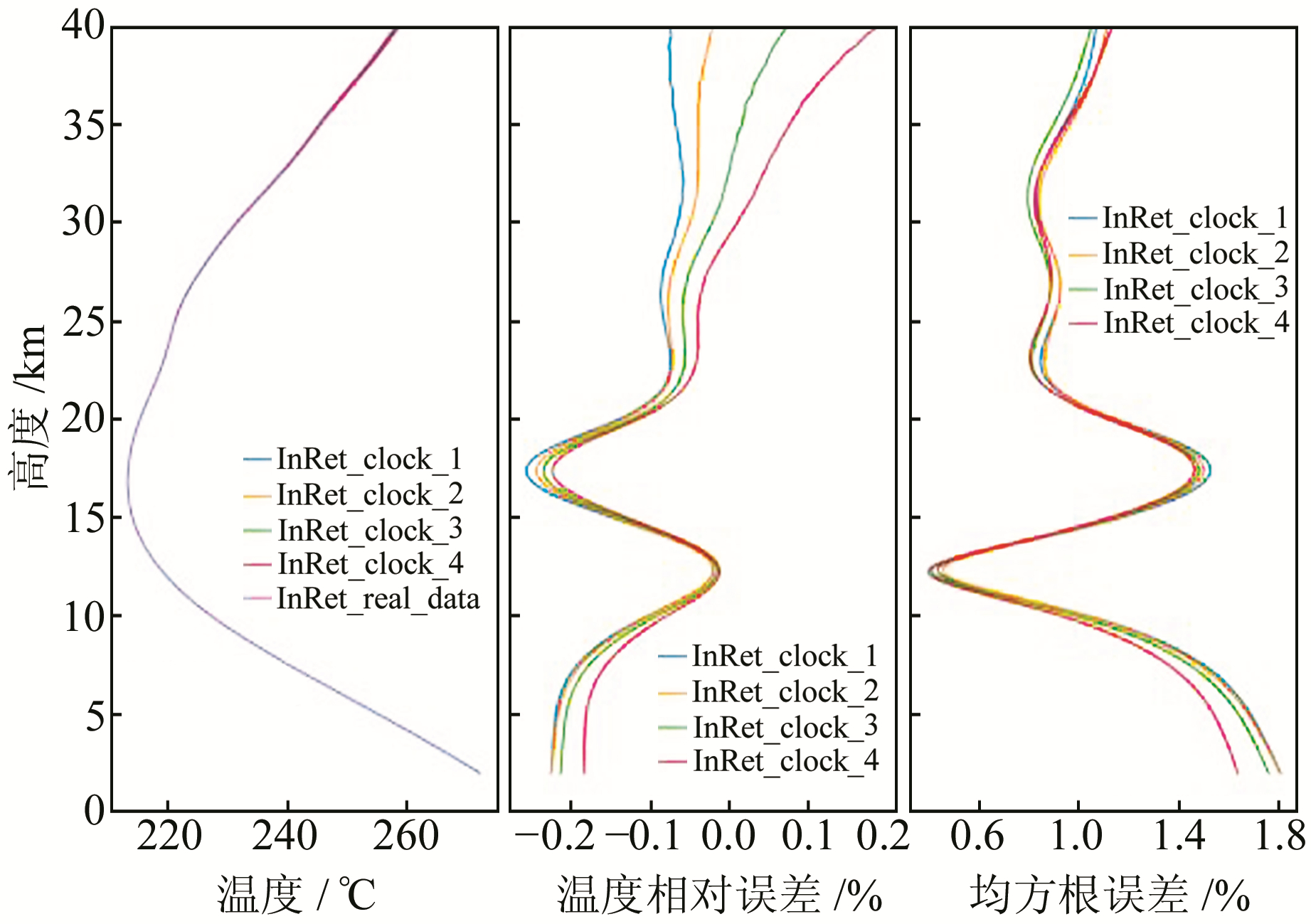图 2 小量级钟稳定性影响统计分析 Fig. 2 Statistical analysis of minor level clock stability表 3 较大量级钟稳定性影响设定及结果 Tab. 3 Larger level clock stability influence settings and results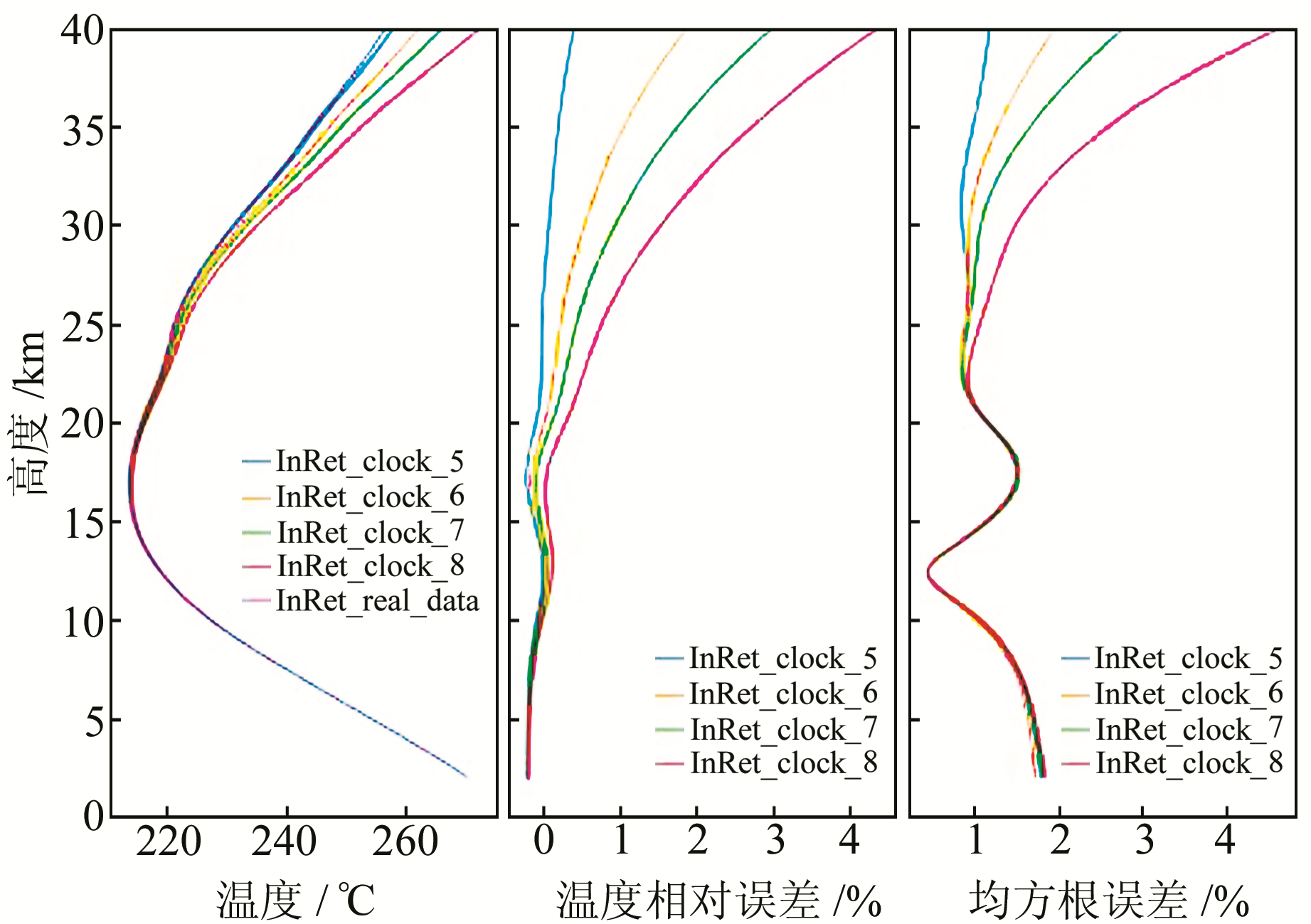图 3 较大量级钟稳定性统计分析 Fig. 3 Statistical analysis of larger level clock stability
3.2 轨道误差

 $\begin{array}{*{20}{c}} {{\rho _1} = }\\ {\sqrt {{{\left( {{X_{{\rm{G1}}}} - {X_{\rm{L}}}} \right)}^2} + {{\left( {{Y_{{\rm{G1}}}} - {Y_{\rm{L}}}} \right)}^2} + {{\left( {{Z_{{\rm{G1}}}} - {Z_{\rm{L}}}} \right)}^2}} } \end{array}$
 $\begin{array}{*{20}{c}} {{\rho _2} = }\\ {\sqrt {{{\left( {{X_{{\rm{G2}}}} - {X_{\rm{L}}}} \right)}^2} + {{\left( {{Y_{{\rm{G2}}}} - {Y_{\rm{L}}}} \right)}^2} + {{\left( {{Z_{{\rm{G2}}}} - {Z_{\rm{L}}}} \right)}^2}} } \end{array}$ (6)

 $\Delta {L_k} = {L_{k2}} - {L_{k1}} = {\rho _2} - {\rho _1}$ (7)表 4 轨道径向位置误差影响设定及结果 Tab. 4 Radial position errors influence settings and results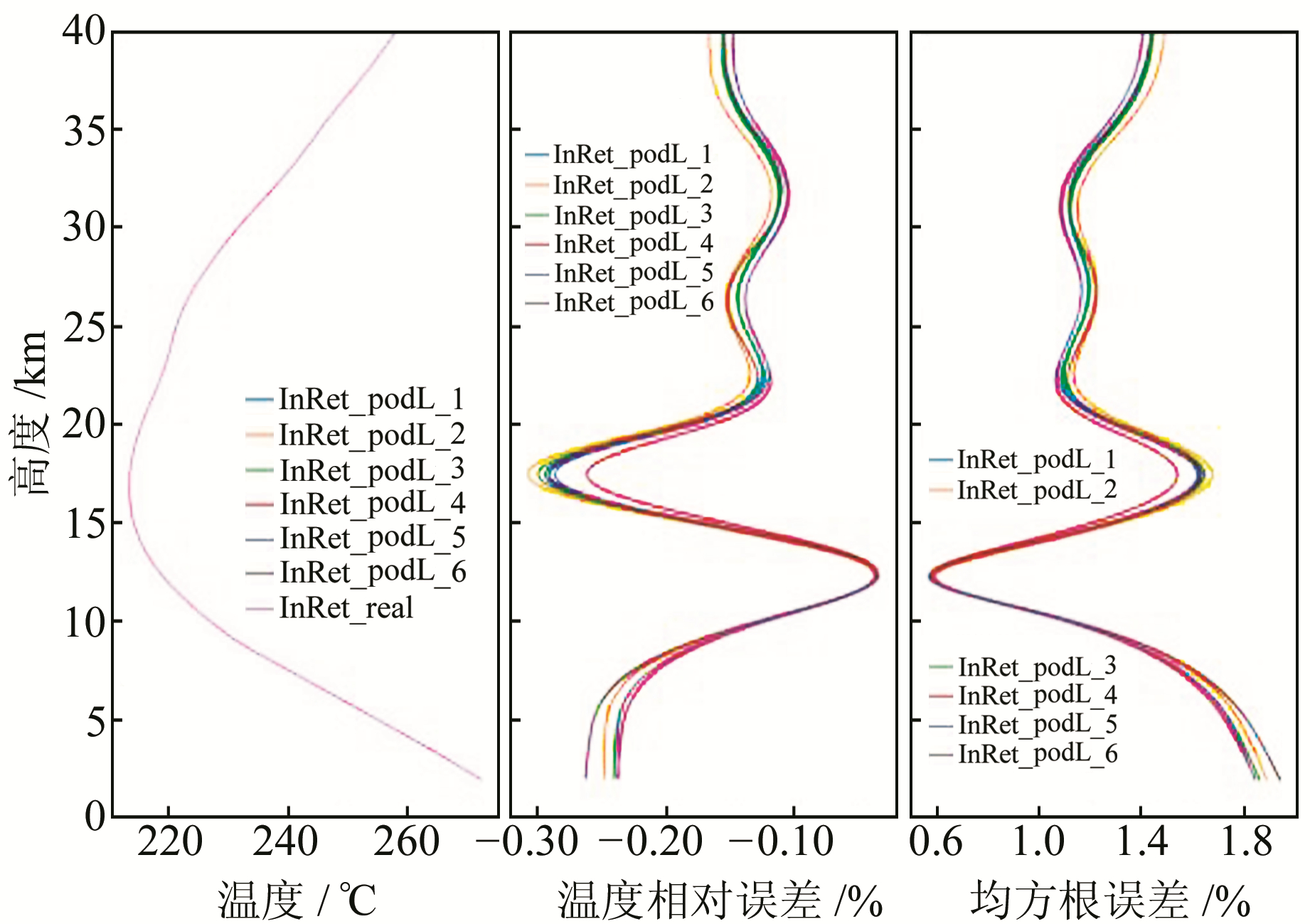图 4 轨道位置误差统计分析 Fig. 4 Statistical analysis of radial position errors表 5 轨道射线误差影响设定及结果 Tab. 5 Along-ray errors influence settings and results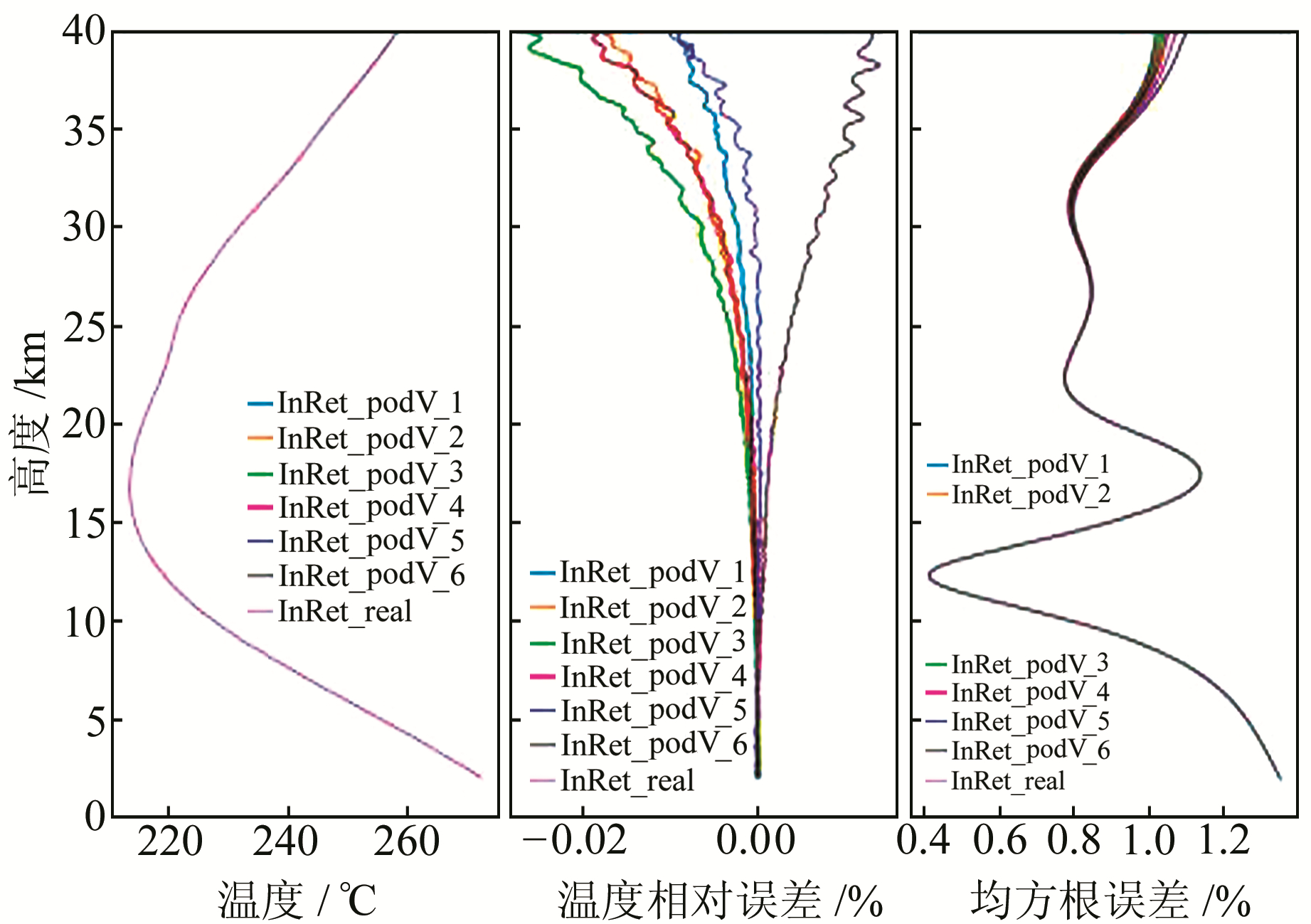图 5 轨道射线误差统计分析 Fig. 5 Statistical analysis of along-ray errors

3.3 噪声误差

 ${\left( {\delta {\varphi ^2}} \right)^{1/2}} = \frac{{\lambda {\sigma _n}\left( \tau \right)}}{{2\pi A}} = \frac{\lambda }{{2\pi }}{\left( {2{\rm{SN}}{{\rm{R}}_0}\tau } \right)^{ - 1/2}}$ (8)表 6 高斯噪声影响设定及结果 Tab. 6 Gaussian phase noise parameters settings and results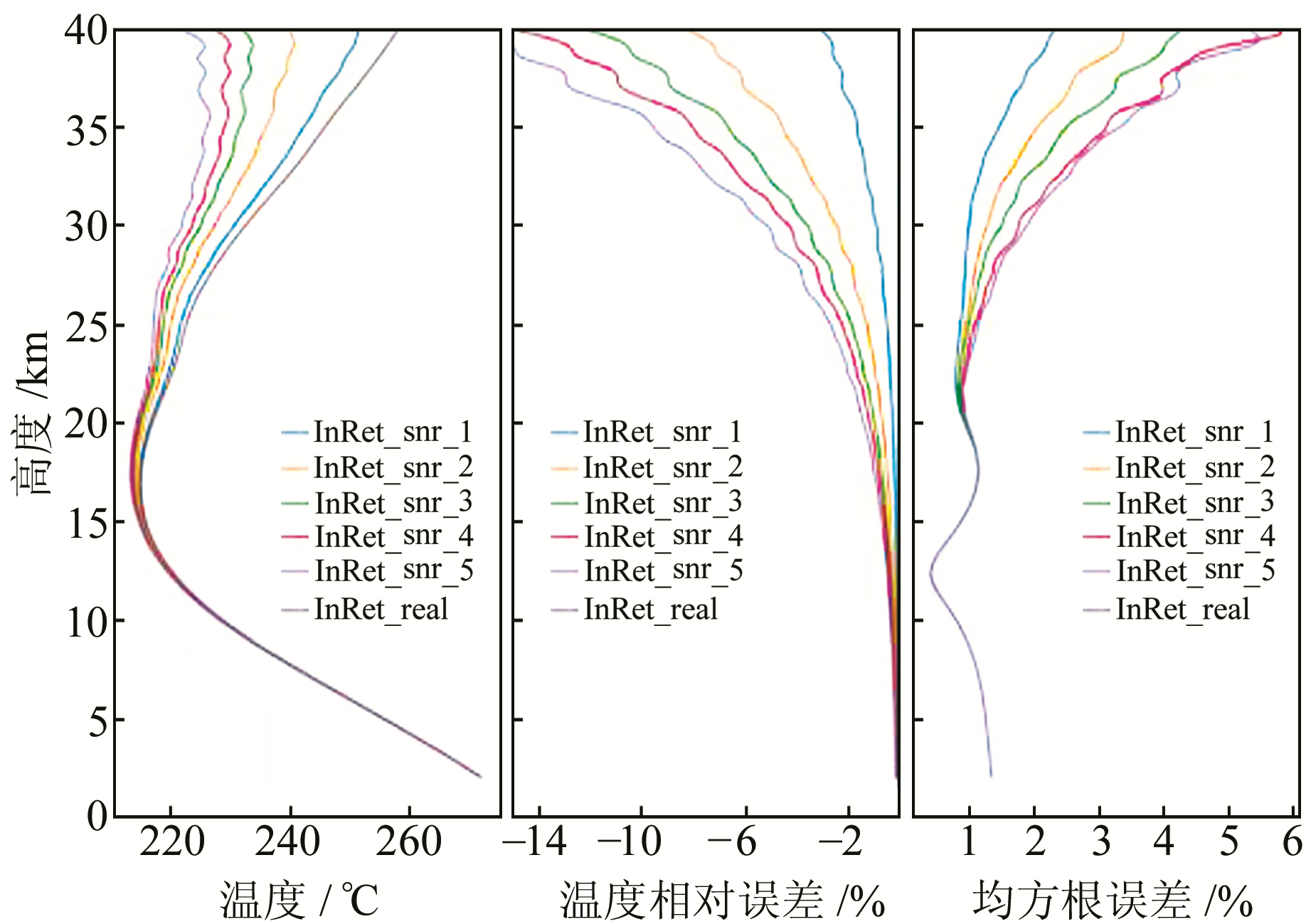图 6 高斯噪声误差统计分析 Fig. 6 Statistical analysis of Gaussian phase noise errors
3.4 局部多路径误差

 ${t_{\sin us}} = {t_{{\rm{begin}}}} - \frac{P}{{2\pi }}{\rm{arcsin}}\left( {\frac{{{A_{{\rm{start}}}}}}{A}} \right)$ (9)
 $\Delta L = A\sin \left[ {\frac{{2\pi }}{P}\left( {{t_{{\rm{ra}}y}} - {t_{\sin us}}} \right)} \right]$ (10)

 $L = A\sin \left[ {\frac{{2\pi }}{P}\left( {{t_{{\rm{ray}}}} - {t_{{\rm{begin}}}}} \right)} \right]$ (11)表 7 局部多路径误差周期影响设定及结果 Tab. 7 Local multipath error period influence settings and results表 8 多路径误差振幅影响设定及结果 Tab. 8 Multipath error amplitude influence settings and results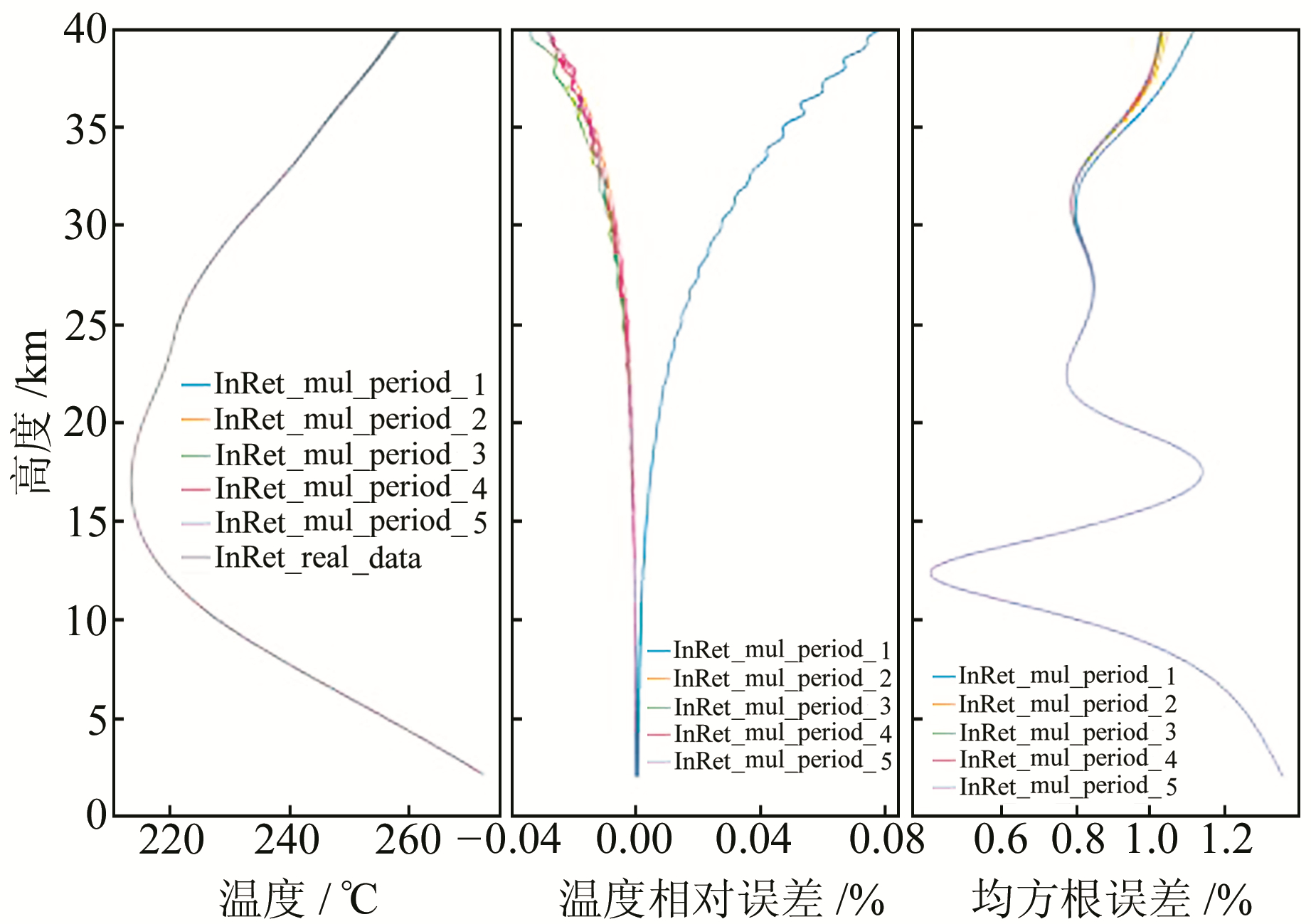图 7 局部多路径误差周期影响统计分析 Fig. 7 Statistical analysis of local multipath error period influence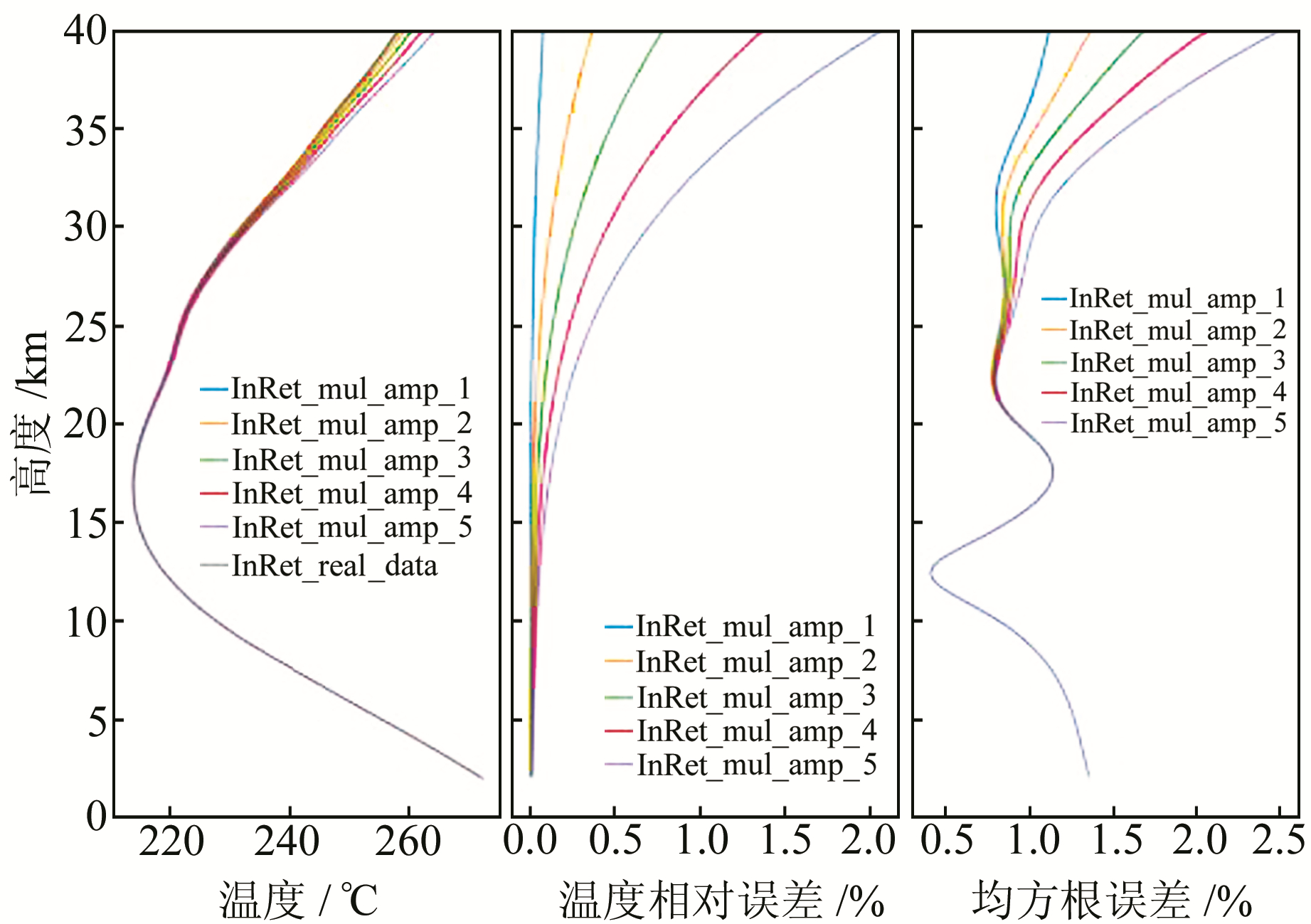图 8 多路径误差振幅影响统计分析 Fig. 8 Statistical analysis of local amplitude error period influence
4 结语

1) 钟的稳定度越高，其反演精度越高。为保证反演精度需求，需将钟稳定性控制在2×10-13以下。

2) 卫星轨道越精确，反演误差越小。现有的卫星cm级的径向位置误差及射线速度误差，可以满足反演需求。

3) 噪声误差对反演精度影响较大，高斯噪声误差在4 mm时，最大相对偏差接近-6%。应当采取其他手段来降低噪声影响，将误差控制在2 mm以内。

4) 多路径误差中，周期影响远小于振幅影响。在20 km以上振幅与温度均方根误差成正相关特性，振幅对中高层大气的反演精度影响较大。

  宫晓艳, 胡雄, 吴小成, 等. 大气掩星反演误差特性初步分析[J]. 地球物理学报, 2007, 50(4): 1017-1029 (Gong Xiaoyan, Hu Xiong, Wu Xiaocheng, et al. Preliminary Analysis of Error Characteristics in Atmospheric Inversion of GPS Radio Occultation[J]. Chinese J Geophys, 2007, 50(4): 1017-1029 DOI:10.3321/j.issn:0001-5733.2007.04.009) (0)  徐晓华, 李征航. GPS气象学研究的最新进展[J]. 黑龙江工程学院学报, 2002, 16(1): 14-18 (Xu Xiaohua, Li Zhenghang. The Advanced Development on the Research of GPS Meteorology[J]. Journal of Heilongjiang Institute of Technology, 2002, 16(1): 14-18 DOI:10.3969/j.issn.1671-4679.2002.01.003) (0)  Beyerle G, Schmidt T, Michalak G, et al. GPS Radio Occultation with GRACE:Atmospheric Profiling Utilizing the Zero Difference Technique[J]. Geophysical Research Letters, 2005, 32(13): 313-320 (0)  Schreiner W, Rocken C, Sokolovskiy S, et al. Estimates of the Precision of GPS Radio Occultations from the COSMIC/FORMOSAT-3 Mission[J]. Geophysical Research Letters, 2007, 34(4): 545-559 (0)  Fjeldbo G, Kliore A J, Eshleman V R. The Neutral Atmosphere of Venus as Studied with the Mariner V Radio Occultation Experiments[J]. Astronomical Journal, 1971, 76: 123-140 DOI:10.1086/111096 (0)  宫晓艳.大气无线电GNSS掩星探测技术研究[D].北京: 中国科学院空间科学与应用研究中心, 2009 (Gong Xiao-yan.Research on GNSS Atmospheric Radio Occultation Technique[D].Beijing: Center for Space Science and Applied Research, CAS, 2009) (0)  Syndergaard S. Retrieval Analysis and Methodologies in Atmospheric Limb Sounding Using the GNSS Radio Occultation Technique[M]. Danish: Danish Meteorological Institute, 1999 (0)  Rees D, Barnett J J, Labitzke K. COSPAR International Reference Atmosphere:1986.Pt.2:Middle Atmosphere Models[J]. Advances in Space Research, 1990, 56(4): 538-539 (0)  Fu N F, Guo P, Wu M J, et al. Retrieval Processing Technique for LEO-LEO Radio Occultation Atmospheric Data and Error Sources Analysis[J]. Remote Sensing Science, 2016, 4(2): 51-64 (0)  Fritzer J, Kirchengast G, Pock M, et al.End-to-End Generic Occultation Performance Simulation and Processing System Version5.5(EGOPS 5.5) Software User Manual[Z].Univ of Graz, Austria, 2010 (0)  Gorbunov M E, Kirchengast G, Lauritsen K B.Advanced Wave-Optics Forward Modeling and Bending Angle and Transmission Retrieval Including Turbulent Random Refractivity Field Modeling[R].Wegener Center, Univ of Graz, Austria, 2006 (0)  Kursinski E R, Hajj G A. The GPS Radio Occulation Technique[J]. TAO, 2000, 11(1): 53-114 (0)
Retrieval on GPS-LEO Atmospheric Radio Occultation and Error Sources Analysis
ZHANG Jiman1,2LIN Jian1,2ZHU Fuying1,2
1. Key Laboratory of Earthquake Geodesy, Institute of Seismology, CEA, 40 Hongshance Road, Wuhan 430071, China;
2. Wuhan Base of Institute of Crustal Dynamics, CEA, 40 Hongshance Road, Wuhan 430071, China
Abstract: Radio occultation technology is an effective means of neutral atmospheric observation. This paper briefly introduces the basic flow of GPS-LEO neutral atmospheric radio occultation inversion. Considering the influence of various error sources on the inversion of atmospheric parameters, we use EGOPS simulation software to simulate 426 occultation events a day using 3D ray tracing method, analyzing the influence characteristics of many error sources. The statistical analysis of the inversion temperature shows that the stability of the satellite clock and the accuracy of the satellite orbit determination can meet the occultation inversion demand. The noise error has a great influence on the inversion, and effective measures are needed. For the multipath effect, the influence of the periodic error is small, but the amplitude has some influence on the inversion result, and it needs to be effectively suppressed.
Key words: radio occultation; error sources analysis; EGOPS